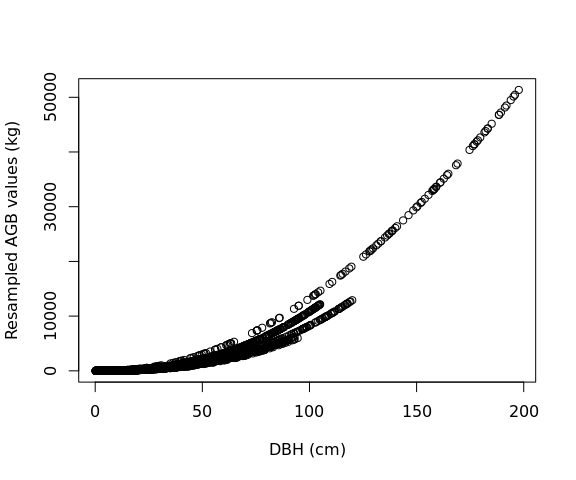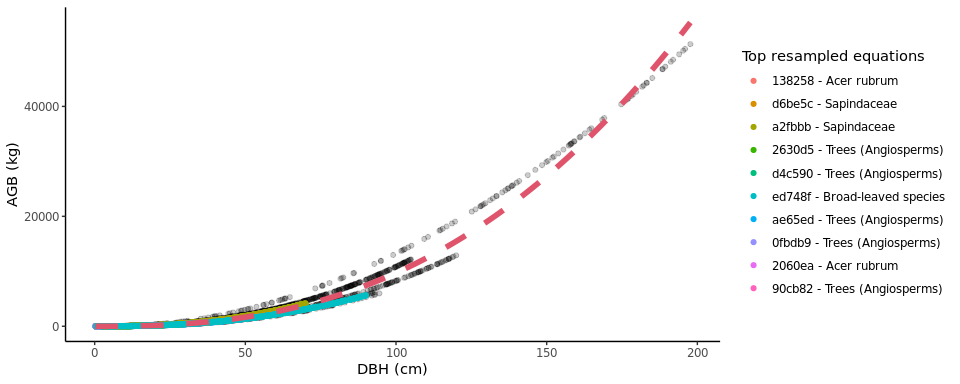## Introduction

Allometric equations for calculation of tree aboveground biomass (AGB) form the basis for estimates of forest carbon storage and exchange with the atmosphere. While standard models exist to calculate forest biomass across the tropics, we lack a standardized tool for computing AGB across the global extratropics.

allodb was conceived as a framework to standardize and simplify the biomass estimation process across globally distributed extratropical forests (mainly temperate and boreal forests). With allodb we aimed to: a) compile relevant published and unpublished allometries, focusing on AGB but structured to handle other variables (e.g., height); b) objectively select and integrate appropriate available equations across the full range of tree sizes; and c) serve as a platform for future updates and expansion to other research sites.

The allodb package contains a dataset of systematically selected published allometric equations. This dataset was built based on 701 woody species identified at 24 large ForestGEO forest dynamic plots representing all major extratropical forest types. A total of 570 parsed allometric equations to estimate individual tree biomass were retrieved, checked, and combined using a weighting function designed to ensure optimal equation selection over the full tree size range with smooth transitions across equations. The equation dataset used can be customized with built-in functions that subset the original dataset and add new equations.

The package provides functions to estimate tree biomass based on user-provided census data (tree diameter, taxonomic identification, and plot coordinates). New allometric equations are calibrated for each species and location by resampling the original equations; equations with a larger sample size and/or higher taxonomic and climatic similarity with the species and location in question are given a higher weight in this process.

## Installation

Install the development version of allodb from GitHub:

``````# install.packages("remotes")
remotes::install_github("ropensci/allodb")``````

## Examples

Prior to calculating tree biomass using allodb, users need to provide a table (i.e. dataframe) with DBH (cm), parsed species Latin names, and site(s) coordinates. In the following examples we use data from the Smithsonian Conservation Biology Institute, USA (SCBI) ForestGEO dynamics plot (trees from 1 hectare surveyed in 2008). Full tree census data can be requested through the ForestGEO portal.

``````library(allodb)
data(scbi_stem1)``````

The biomass of all trees in one (or several) censuses can be estimated using the `get_biomass` function.

``````scbi_stem1\$agb <-
get_biomass(
dbh = scbi_stem1\$dbh,
genus = scbi_stem1\$genus,
species = scbi_stem1\$species,
coords = c(-78.2, 38.9)
)``````

Biomass for a single tree can be estimated given dbh and species identification (results in kilograms).

``````get_biomass(
dbh = 50,
genus = "liriodendron",
species = "tulipifera",
coords = c(-78.2, 38.9)
)
#>  1578.644``````

Users can modify the set of equations that will be used to estimate the biomass using the `new_equations` function. The default option is the entire allodb equation table. Users can also work on a subset of those equations, or add new equations to the table (see `?allodb::new_equations`). This new equation table should be provided as an argument in the `get_biomass` function.

``````show_cols <- c("equation_id", "equation_taxa", "equation_allometry")
eq_tab_acer <- new_equations(subset_taxa = "Acer")
#> # A tibble: 6 × 3
#>   equation_id equation_taxa       equation_allometry
#>   <chr>       <chr>               <chr>
#> 1 a4e4d1      Acer saccharum      exp(-2.192-0.011*dbh+2.67*(log(dbh)))
#> 2 dfc2c7      Acer rubrum         2.02338*(dbh^2)^1.27612
#> 3 eac63e      Acer rubrum         5.2879*(dbh^2)^1.07581
#> 4 f49bcb      Acer pseudoplatanus exp(-5.644074+(2.5189*(log(pi*dbh))))
#> 5 14bf3d      Acer mandshuricum   0.0335*(dbh)^1.606+0.0026*(dbh)^3.323+0.1222*…
#> 6 0c7cd6      Acer mono           0.0202*(dbh)^1.810+0.0111*(dbh)^2.740+0.1156*…``````

Within the `get_biomass` function, this equation table is used to calibrate a new allometric equation for all species/site combinations in the user-provided dataframe. This is done by attributing a weight to each equation based on its sampling size, and taxonomic and climatic similarity with the species/site combination considered.

``````allom_weights <-
weight_allom(
genus = "Acer",
species = "rubrum",
coords = c(-78, 38)
)

## visualize weights
equ_tab_acer <- new_equations()
equ_tab_acer\$weights <- allom_weights
keep_cols <-
c(
"equation_id",
"equation_taxa",
"sample_size",
"weights"
)
order_weights <- order(equ_tab_acer\$weights, decreasing = TRUE)
equ_tab_acer <- equ_tab_acer[order_weights, keep_cols]
#> # A tibble: 6 × 4
#>   equation_id equation_taxa        sample_size weights
#>   <chr>       <chr>                      <dbl>   <dbl>
#> 1 138258      Acer rubrum                  150   0.415
#> 2 d6be5c      Sapindaceae                  243   0.383
#> 3 a2fbbb      Sapindaceae                  200   0.349
#> 4 2630d5      Trees (Angiosperms)          886   0.299
#> 5 d4c590      Trees (Angiosperms)          549   0.289
#> 6 ed748f      Broad-leaved species        2223   0.270``````

Equations are then resampled within their original DBH range: the number of resampled values for each equation is proportional to its weight (as attributed by the `weight_allom` function).

``````df_resample <-
resample_agb(
genus = "Acer",
species = "rubrum",
coords = c(-78, 38)
)

plot(
df_resample\$dbh,
df_resample\$agb,
xlab = "DBH (cm)",
ylab = "Resampled AGB values (kg)"
)``````The resampled values are then used to fit the following nonlinear model:, with i.i.d.. The parameters (a, b, and sigma) are returned by the `est_params()` function.

The resampled values (dots) and new fitted equation (red dotted line) can be visualized with the `illustrate_allodb()` function.

``````pars_acer <- est_params(
genus = "Acer",
species = "rubrum",
coords = c(-78, 38)
)
illustrate_allodb(
genus = "Acer",
species = "rubrum",
coords = c(-78, 38)
)``````The `est_params` function can be used for all species/site combinations in the dataset at once.

``````params <- est_params(
genus = scbi_stem1\$genus,
species = scbi_stem1\$species,
coords = c(-78.2, 38.9)
)
AGB is then recalculated as `agb = a * dbh^b` within the `get_biomass` function.